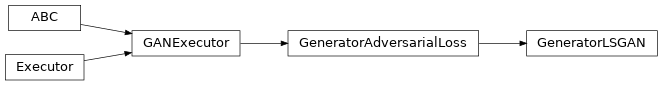GeneratorLSGAN¶

Inheritance Diagramclass ashpy.losses.gan.GeneratorLSGAN[source]

Least Square GAN Loss for generator.

Reference: https://arxiv.org/abs/1611.04076

Note

Basically the Mean Squared Error between the discriminator output when evaluated in fake and 1.

$L_{G} = \frac{1}{2} E [(1 - D(G(z))^2]$

Methods

 __init__() Initialize the Least Square Loss for the Generator.

Attributes

 fn Return the Keras loss function to execute. global_batch_size Global batch size comprises the batch size for each cpu. weight Return the loss weight.
__init__()[source]

Initialize the Least Square Loss for the Generator.

Return type: None